# Brief Review — A Simple Way to Initialize Recurrent Networks of Rectified Linear Units

## IRNN, RNN Initialized Using Identity Matrix

A Simple Way to Initialize Recurrent Networks of Rectified Linear Units
2015 arXiv v2, Over 700 Citations (Sik-Ho Tsang @ Medium)
Recurrent Neural Network, RNN

• To overcome vanishing and exploding gradients, instead of using LSTM, identity matrix or its scaled version is proposed to initialize the recurrent weight matrix. This is a tech report from Prof. Hinton’s research group.

# Outline

1. RNN Initialized Using Identity Matrix (IRNN)
2. Results

# 1. RNN Initialized Using Identity Matrix (IRNN)

## 1.1. Identity Matrix

• The standard RNN uses ReLU instead of Tanh.
• Gradient clipping, used in SGD+CR, is used.
• The recurrent weight matrix is initialized to be the identity matrix and biases to be zero, such that no extra error-derivatives are added at the beginning.

## 1.2. Scaled Version

• For tasks that exhibit less long range dependencies, scaling the identity matrix by a small scalar is an effective mechanism to forget long range effects.

# 2. Results

## 2.1. Adding Problem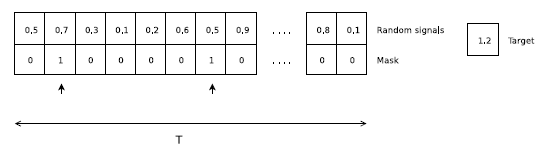An example of the “adding” problem, where the target is 1.2 which is the sum of 2nd and the 7th numbers in the first sequence
• At every time step, the input consists of a random signal and a mask signal.
• This is a sequence regression problem where the target is a sum of two numbers selected in a sequence of random signals.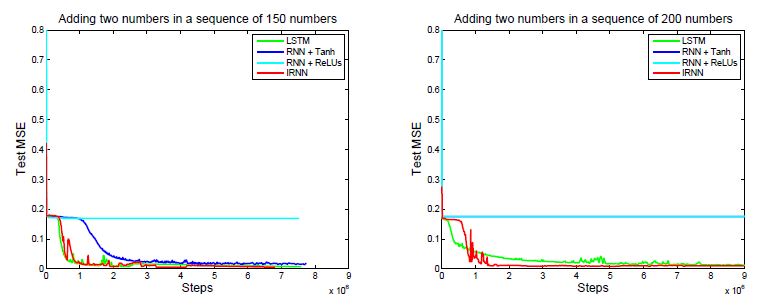The results of recurrent methods on the “adding” problem for the case of T = 150 (left), T = 200 (right)

The convergence of IRNNs is as good as LSTMs. Yet, each LSTM step is more expensive than an IRNN step.

## 2.2. MNIST Classification from a Sequence of Pixels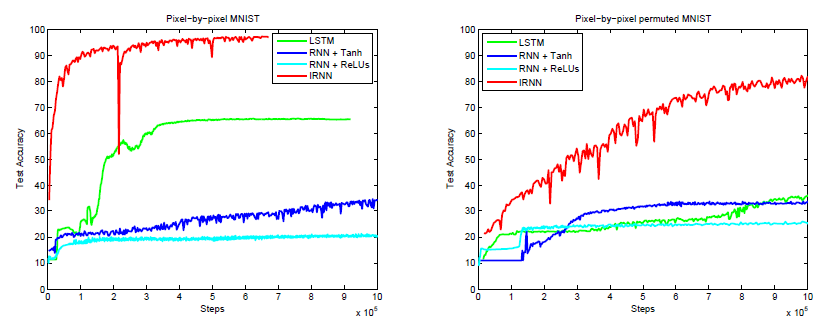The results of recurrent methods on the “pixel-by-pixel MNIST” problem
• The networks read one pixel at a time in scanline order (i.e. starting at the top left corner of the image, and ending at the bottom right corner). This is therefore a huge long range dependency problem because each recurrent network has 784 time steps.
• All networks have 100 recurrent hidden units.

Standard RNNs fail to work, even with ReLUs, whereas the IRNN achieves 3% test error rate. LSTM did not work as well as IRNN.

## 2.3. Language Model on 1 Billion Word Benchmark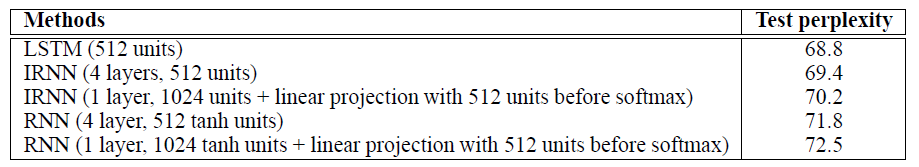Performances of recurrent methods on the 1 billion word benchmark

The performance of IRNNs is closer to the performance of LSTMs for this large-scale task than it is to the performance of RNNs.

## 2.4. Frame Error Rate on TIMIT Phone Recognition Task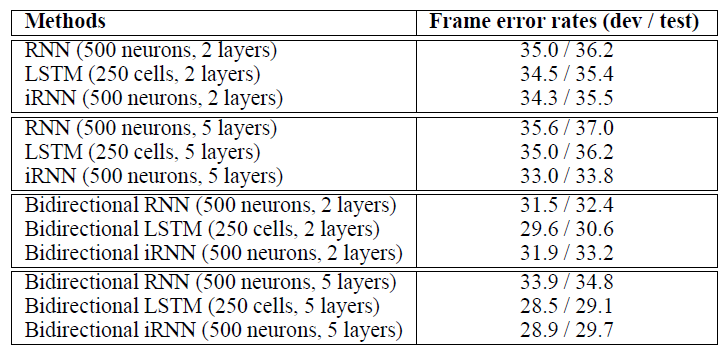Frame error rates of recurrent methods on the TIMIT phone recognition task
• Scaled value of 0.01I is used in IRNN.

The IRNN easily outperforms the RNN that uses tanh units and is comparable to LSTM.

Identity matrix or its scaled version is used for weight initialization to stabilize the training and achieve better performance.

## Reference

[2015 arXiv v2] [IRNN]
A Simple Way to Initialize Recurrent Networks of Rectified Linear Units

## Language Model / Sequence Model

2007 2015 … [IRNN] 2016 … 2020 [ALBERT] [GPT-3] [T5] [Pre-LN Transformer] [MobileBERT] [TinyBERT]

--

--